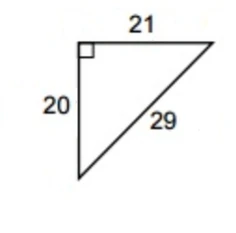## Problem

There are two persons standing at the same location. Person A moves at a speed of 7. Person B moves at a speed of 3. Person B moves east. Person A first moves 10 bu south, then diagonally northeast until he meets person B once more. How far did each man travel?

Note: The units of the speed is not specified. Assume that the speed is in bu/second. The Chinese unit of length bu (步) literally means pace, and it measures roughly 1.6 meters .

## Solution

Here is the solution found in the Jiuzhang Suanshu.

Answer: Person B travels 10.5 bu; person A travels 14.5 bu diagonally.

Method: Halve the sum of 7 squared and 3 squared, and let this represent the diagonal ratio traveled by person A. Subtract the diagonal distance ratio from 7 squared to obtain the southern ratio. Multiply 3 with 7 to obtain the eastern ratio traveled by person B. Set the southern distance of 10 bu, and multiply with person A's diagonal ratio, set 10 bu and multiply with person B's eastern distance rate, and treat both as shi. Divide each shi by the southern ratio to obtain the respective distances.

This problem is an application of Pythagorean triples. First compute the rates of the height, base, and hypotenuse. According to the method the height, base, hypotenuse are called the southern ratio, the eastern ratio, and the diagonal ratio respectively. Let, andGiven that person A traveled 10 bu due south before travelling diagonally, the distance traveled by person B is.

The diagonal distance travelled by person A is.

Thus person A travelled 24.5 bu, and person B travelled 10.5 bu.

For a derivation of why this method works, check out this page.